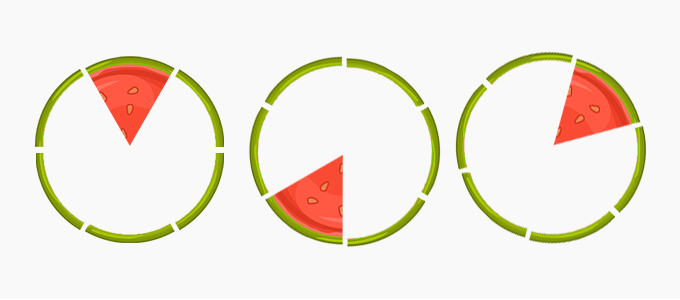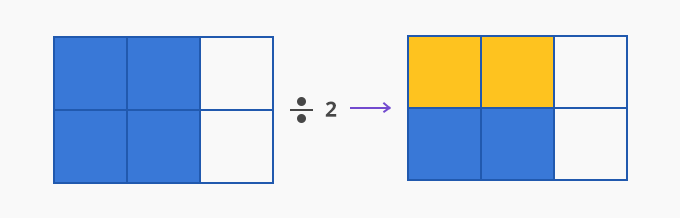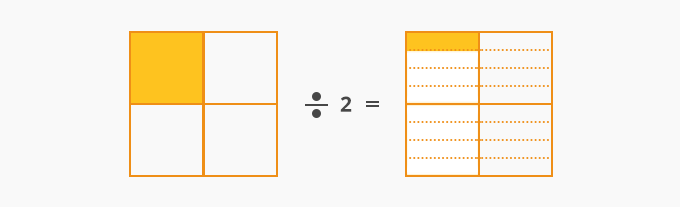# Dividing Fractions With Whole Numbers - Definition with Examples

The Complete K-5 Math Learning Program Built for Your Child

• 40 Million Kids

Loved by kids and parent worldwide

• 50,000 Schools

Trusted by teachers across schools

• Comprehensive Curriculum

Aligned to Common Core

## Dividing Fractions with Whole Numbers

A fraction is a part of a whole. The given pizza is cut into 5 equal slices and 3 of slices are left. That is, 3 out of 5 slices of pizza are there. The fraction shown is 35Now, if we divide this three-fifths of the pizza into 3 equal parts, each part will have one part out of the 5 parts as shown.That is 35÷3= 1⁄5.

Dividing 35 by 3, will give us one-third of 35

That is 3115.

We can also verify this as  1x 3= 35.

Consider dividing the fraction 46 by 2.The portion shaded in pink is divided equally into two parts – shaded in green and the one in blue respectively. The part in green is 36 of the rectangle, and so is the part shaded in blue.

That is 462=26.

We can verify this using multiplication as  2x 2 = 46.

Here again, on dividing 46 by 2, we precisely find the one-half of 46

That is 41226.

In both the examples, in the procedure, the division symbol is replaced with multiplication, and its multiplicative inverse or reciprocal replaces the divisor.

The rule is, to divide a fraction by a whole number, multiply the given fraction by the reciprocal of whole numbers.

Example: Find  14÷3.

The reciprocal of 3 is 13

14÷3 = 11112

Conceptually this can be shown as:Example: If 5 out of 12 pieces of an apple pie were shared among 3 people, what fraction of apple pie does each person gets?

We know that 512 of the apple pie is equally shared between 3 people.

So, we have to find 512 ÷3.

The reciprocal of 3 is 13

512 ÷ 3 = 512 1536

Therefore, each person gets 536 of the apple pie.

Fun Facts:

What if the divisor is a fraction? The rule remains the same!

3÷ 14 = 3x 4 = 3

How many ¼ s are there in a ¾?

There are 3 one-fourth in a three-fourth!

• If the dividend is a mixed fraction, first convert the mixed fraction into an improper fraction and apply the rule.

Example: 51÷ 8

First, convert 513 into an improper fraction.

51= (5x3)+1163

Now, 51÷ 8 = 163 ÷ 8 = 163 x 18 = 23 .

Won Numerous Awards & Honors